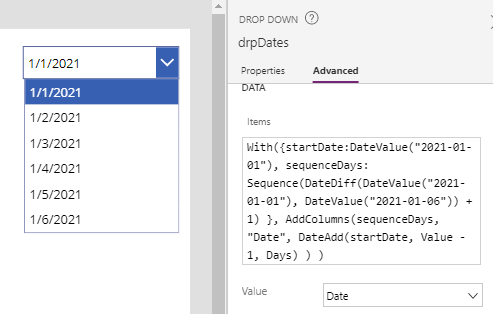# Formulas - how to return all days between two dates

There are some scenarios where we need to return all the days between a given start and end date. This post describes the formula to build a table of sequential, consecutive days.

There are often scenarios where we need to display, or to build a collection that contains a range of sequential dates.

As an example, let's take a data entry screen in an app that manages multi-day events or conferences. On this screen, we may want to display a drop-down box with a choice of values between a start and end date.

Alternatively, let's imagine an app that bulk inserts multiple records. To implement this type of feature, we may want to base the functionality on a collection with records that correspond to each day between the start and end date values.

## How to show consecutive days in a control (eg, a drop down)

The formula beneath shows the basic syntax that we would use. This formula produces an output table that contains all dates between the "1st January 2021" and "6th January 2021" inclusively.

`With({startDate:DateValue("2021-01-01"),      sequenceDays: Sequence(DateDiff(DateValue("2021-01-01"),                                      DateValue("2021-01-06")) + 1)      },      AddColumns(sequenceDays,                 "Date",                 DateAdd(startDate, Value - 1, Days)       ))`

To apply this formula, we would replace the start date and end date values inside the With block, with our target start and end dates..

This formula relies on a single column table called sequenceDays. This table contains a consecutive sequence of numbers between the start and end dates. In this example, the content of the sequenceDays collection would contain the following data:

`[1,2,3,4,5,6]`

We use a combination of the Sequence and DateDiff functions to generate the sequenceDays collection. In this example, DateDiff returns the number of days between the start and end date which is 5. To produce an output that is inclusive of both the start and end dates, we need to add 1 to this value.

We add a column called "Date", which we calculate by adding the sequence value to the start date. Because the sequence begins with 1 and we want to add zero days for the first output record, we subtract the 'value' in the sequence by 1.

The screenshot beneath illustrates this technique, as applied to the Items property of a drop down control.## How to populate a collection of consecutive days with additional fields

As a logical extension of the above, here's the formula to populate a collection with the same days, but with extra columns to display the day numbers.

`With({startDate:DateValue("2021-01-01"),      sequenceDays: Sequence(DateDiff(DateValue("2021-01-01"),                                      DateValue("2021-01-06")) + 1)      },      ClearCollect(colDates,                    AddColumns(sequenceDays,                              "Date",                               DateAdd(startDate, Value - 1, Days),                              "Day Num",                              "Day " & (Value),                              "Day Name",                                                            Text(DateAdd(startDate, Value - 1, Days),                                   "dddd"                              )                   )      ))`
We implement this by defining the fields the columns to add in the call to AddColumns. Notice how we apply the a technique from an earlier post to display the day name (eg, Monday, Tuesday, etc)

The screenshot beneath illustrates the appearance of a data table, when we apply the Items property to the collection.

## Conclusion

A common requirement is to build a collection of sequential days between the start and end date value. This post highlighted how to accomplish this with the help of the Sequence, DateDiff, and AddColumn functions.